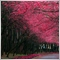• Overview
• Reviews (3)
• What's new

# PZ Pivot Points MT5

This indicator displays pivot points in the chart, including historical vales, and supports many calculation modes for Pivot Points and S/R levels. [ Installation Guide | Update Guide | Troubleshooting | FAQ | All Products ]

• It plots historical levels for backtesting purposes
• It allows you to select the reference timeframe
• It implements different Pivot Point calculation modes
• It implements different SR calculation modes
• It implements customizable colors and sizes

### Calculation Modes

The indicator implements different calculation modes for the pivot point.
• (H+L+C)/3
• (H+L+O)/3
• (H+L+C+O)/4
• (H+L+C+C)/4
• (H+L+O+O)/4
• (H+L)/2
• (H+C)/2
• (L+C)/2

It also implements many different calculation modes for SR Levels:

1. Classic Formula
• R4 = R3 + (High – Low)
• R3 = R2 + (High – Low)
• R2 = PP + (High – Low)
• R1 = (2 * PP) – Low
• S1 = (2 * PP) – High
• S2 = PP – (High – Low)
• S3 = S2 – (High – Low)
• S4 = S3 – (High – Low)

2. Woodie Pivot Points
• R4 = R3 + (High – Low)
• R3 = High + 2 * (PP – Low)
• R2 = PP + (High – Low)
• R1 = (2 * PP) – Low
• S1 = (2 * PP) – HIGH
• S2 = PP – (High – Low)
• S3 = Low – 2 * (High – PP)
• S4 = S3 – (High – Low)

3. Camarilla Pivot Points
• R4 = PP + (High – Low) * 1.1/2
• R3 = PP + (High – Low) * 1.1/4
• R2 = PP + (High – Low) * 1.1/6
• R1 = PP + (High – Low) * 1.1/12
• S1 = PP – (High – Low) * 1.1/12
• S2 = PP – (High – Low) * 1.1/6
• S3 = PP – (High – Low) * 1.1/4
• S4 = PP – (High – Low) * 1.1/2

### Input Parameters

• PP Calculation Mode: Select the Pivot Points calculation mode desired
• S/R Calculation Mode: Select the SR calculation mode desired
• Timeframe: Choose the timeframe to calculate pivot points from
• Mid Points: Display or hide mid points between SR levels
• Color of S levels: Color of support levels
• Color of R levels: Color of resistance levels
• Color of P level: Color of pivot point levels
• Font size: Font size for name and price labels

### Support

I am happy to provide free products but given my limited time, they come without support of any kind. My apologies.

### Author

Arturo López Pérez, private investor and speculator, software engineer and founder of Point Zero Trading Solutions.1249
2020.05.15 20:37

&gt;Good . Thanks fro free!!15
2020.05.09 00:59